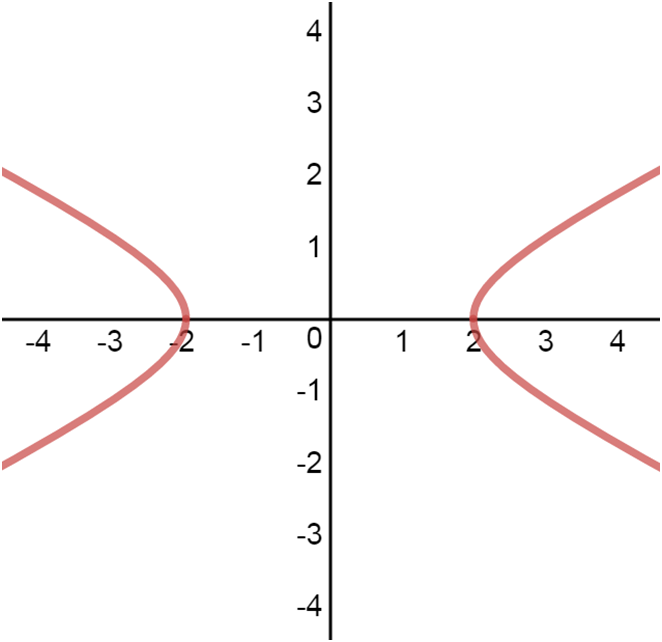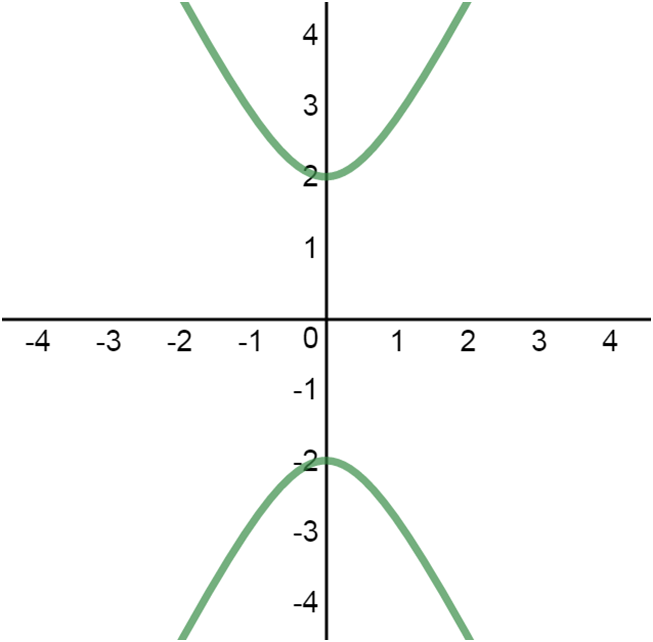# Conics - Hyperbola#### Everything You Need in One Place

Homework problems? Exam preparation? Trying to grasp a concept or just brushing up the basics? Our extensive help & practice library have got you covered.#### Learn and Practice With Ease

Our proven video lessons ease you through problems quickly, and you get tonnes of friendly practice on questions that trip students up on tests and finals.#### Instant and Unlimited Help

Our personalized learning platform enables you to instantly find the exact walkthrough to your specific type of question. Activate unlimited help now!

0/1
##### Intros
###### Lessons
1. Hyperbola:
horizontal hyperbolavertical hyperbola• Graph looks sort of like two mirrored parabolas, with the two "halves" being called "branches".
• "Vertices" are defined similarly to the way of a "vertex" is defined for a parabola.
• Just as the focus for a parabola, the two foci for a hyperbola are inside each branch.
• The line connecting the two vertices is called the "transverse axis".
0/8
##### Examples
###### Lessons
1. properties of a hyperbola
$-(\frac{x-6}{4})^2 + (\frac{y+5}{3})^2=1$
1. Identify the type of conic section.
2. State the "center".
3. Set up the guidelines for the conic graph.
4. Find the "vertices".
5. Locate the "foci".
6. Find the "eccentricity".
7. Find the equations of the "asymptotes".
8. Find the lengths of transverse axis and conjugate axis.
###### Topic Notes
hyperbola: the difference of the distances from any point on a hyperbola to each focus is constant and equal to the transverse axis $2a$.
ellipse: the sum of the distances from any point on an ellipse to each focus is constant and equal to the major axis $2a$.

$c= \sqrt{a^2 - b^2}$ $c$: distance from the center to a focus

$e= \frac{c}{a}$ $e$: eccentricity; the larger the value of $e$, the straighter the hyperbola